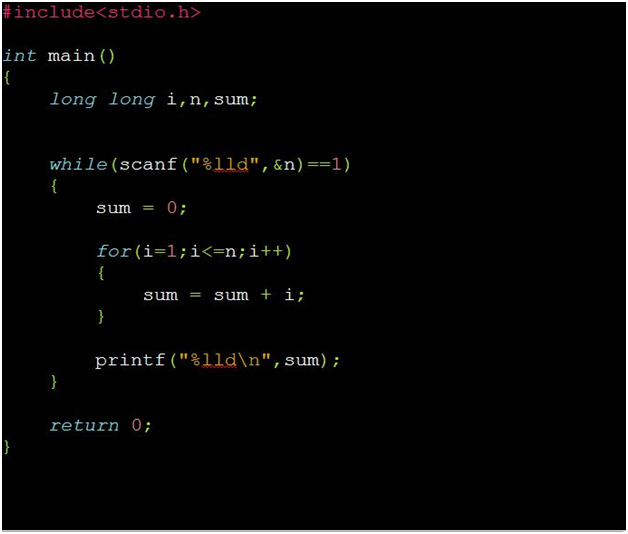Problems
10073 - Convert The Code

Convert The Code

Time Limit: 0.5 sec

The Problem

Arafat is new to programming. He found a problem in an OJ (online judge) and solved it. But after submitting the answer he got TLE (time limit exceeded). But he could not find what the problem in his code is. Help him solving the problem. His code is given below:The Input

There will be an integer N (0<=N<=1000000000) in each test case.  Input will be terminated by EOF (end of file).

The Output

For each test case print a single integer, the answer of the problem according to the given code.

Sample Input

1

12

100

Sample Output

1

78

5050

Aracari contest [School & College Level]

Problem Setter: Fahim Abrar